# RS Aggarwal Class 7 Solutions Chapter 11 Profit and Loss Ex 11B

## RS Aggarwal Class 7 Solutions Chapter 11 Profit and Loss Ex 11B

These Solutions are part of RS Aggarwal Solutions Class 7. Here we have given RS Aggarwal Solutions Class 7 Chapter 11 Profit and Loss Ex 11B.

Other Exercises

Objective Questions :
Mark (✓) against the correct answer in each of the following :
Question 1.
Solution:
(b)
C.P. = Rs. 80
S.P. = Rs. 100
Gain = S.P. – C.P. = Rs. 100 – 80 = Rs. 20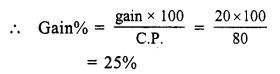Question 2.
Solution:
(a)
C.P. = Rs. 120
S.P. = Rs. 105
Loss = C.P. – S.P. = Rs. 120 – 105 = Rs. 15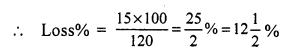Question 3.
Solution:
(b)
S.P. = Rs. 100
Gain = Rs. 20
C.P. = S.P. – gain = Rs. 100 – 20 = Rs. 80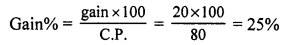Question 4.
Solution:
(a)
S.P. = Rs. 198
Gain = 10%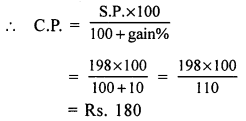Question 5.
Solution:
(a)
First S.P. of jug = Rs. 144
Loss = $$\frac { 1 }{ 7 }$$ of C.P.
Let C.P. = x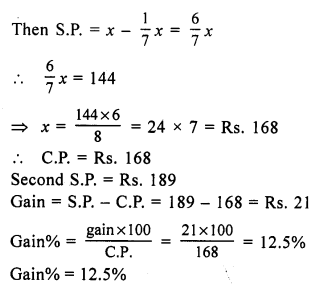Question 6.
Solution:
(d)
First S.P. of a pen = Rs. 48
Loss = 20%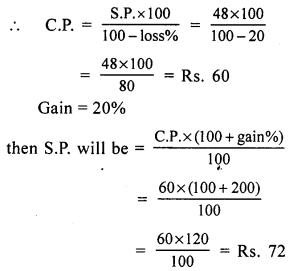Question 7.
Solution:
(a)
C.P. of 12 pencils = S.P. of 15 pencils = Re 1 (suppose)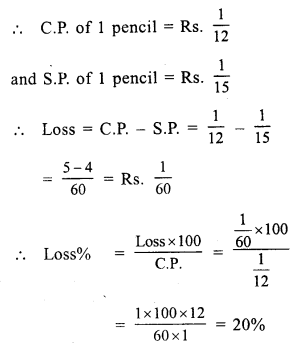Question 8.
Solution:
(d)
Let CP of 4 toffees = SP of 3 toffee = Rs. 12
(LCM of 4, 3 = 12)
CP of 1 toffee = Rs. $$\frac { 12 }{ 4 }$$ = Rs. 3
SP of 1 toffee = Rs. $$\frac { 12 }{ 3 }$$ = 4
Gain = Rs. 4 – 3 = Re 1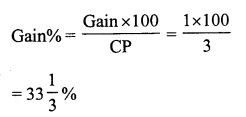Question 9.
Solution:
(c) SP of an article = Rs. 144
Loss% = 10%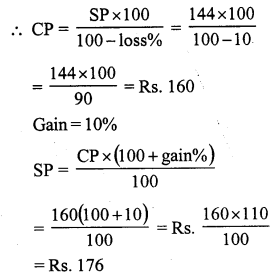Question 10.
Solution:
(a)
CP of 6 lemons = Re 1
CP of 1 lemon = Re $$\frac { 1 }{ 6 }$$
SP of 4 lemons = Re 1
SP of 1 lemon = Re $$\frac { 1 }{ 4 }$$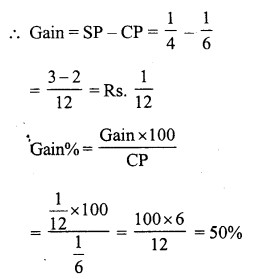Question 11.
Solution:
(d)
SP of chair = Rs. 720
Gain% = 20%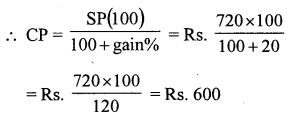Question 12.
Solution:
(c)
SP of stool = Rs. 630
Loss% = 10%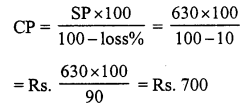Hope given RS Aggarwal Solutions Class 7 Chapter 11 Profit and Loss Ex 11B are helpful to complete your math homework.

If you have any doubts, please comment below. Learn Insta try to provide online math tutoring for you.